Username Password Are you new? Sign up now I’ve forgotten Home :. Downloads :. Articles :. Projects :. Reviews :. Forums :. Arcade :. Klikcast :. GOTW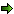Post ReplyPost Oekaki

 Posted By Messagebhlaab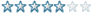Registered  19/06/2002 Points  73 13th December, 2006 at 18:21:01 - Okay this enemy is tossing a grenade. I want it to arc up and come down where my character is standing even if the enemy is situated a higher or lower level. I'm just not that good at math and the formulas involved. So far I've managed to get it to work pretty well while on the same level just using a pinball movement and adjusting the speed and gravity based on the distance between the enemy and the player, however putting the enemy on a higher or lower level completely bungs everything up with my current not-very-mathematic code. Please help with this it's driving me insane.   n/aJoe.HEvil Faker Registered  19/08/2002 Points  3305 14th December, 2006 at 10:28:22 - Distance between points: sqrrt((x1-x2)^2 + (y1-y2)^2) You're best off using a sin function for the movement of the grenade (Move it parallel to the imaginary line between 2 points) Sin will take values between 0 and 1 when 0 <= angle <= 180 So basically you need to increase the angle relative to the line (it hits 90 degrees at the mid point, and 180 at the end of it, i.e it's target) Normally it would be (some condition) = Set Y position of grenade to Y position of throw - H * sin(Alterable Value A("Active")) In ths case if you multiply it by something you can increase the height it moves by (SF H) That's for a straight horizontal surface not sure about an angled surface, you might need to multiply by the sine or cosine of the angle of the line between the player and the target i.e Sin(Angle of grenade) * (tan ((Y2-Y1)/(X2-X1)) Horizontal force is define as Fsin(angle) where F is the force it is hit with Vertical resultant force is R = Fcos(angle) + mg (m = mass g = 9.81) However, mg can be neglected unless you're doing proper physics Basically the Fcos(angle) would be how fast it would move along that point. So if you want to move vertically you'd do Y ("Grenade") - (an alterable value("Grenade") * Fcos(angle)) The alterable value A should be 0 initially, increase to something for half it's path, go to 0 at this point and then start decreasing below 0, so it moves down Same for X, but only times by the sin of the angle I think this is right Probably not though, even though i do physics and mathsMy signature is never too big!!!axelCrazy? Registered  05/02/2005 Points  4766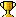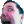14th December, 2006 at 12:09:25 - Wtf you're a math leet. But keep in mind that all those arithmetic operators are a bit different in MMF. Pow is power, Sqr() is square root.   n/a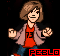PebloCustom ratings must be 50 characters or lessRegistered  05/07/2002 Points  185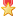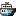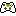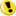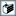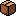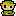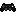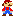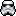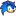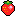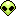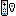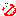14th December, 2006 at 12:10:37 - Dude the grenade will never hit if the player is underground.   "Isn't it always amazing how we characterize a person's intelligence by how closely their thinking matches ours?" ~BelgarathNoyb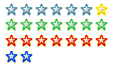Registered  31/05/2004 Points  111714th December, 2006 at 18:50:38 - x - x0 = vt + .5at^2 is a basic physics formula for 1-dimensional movement. v = velocity (pixels/loop), t = time (loops), a = acceleration (pixels/loop^2). We can use this equation twice, one for each of the axes the grenade will move. To make it simple, presume you want the grenade to always hit its target at a predefined, constant t. For horizontal movement, there is no acceleration (unless you want to factor in wind/air resistance, in which case you would use equations similar to the vertical movement). So the initial Xvelocity = (xPlayer - xGrenade) / t. For vertical movement, you need to factor in the acceleration due to gravity. So the initial Yvelocity = (xPlayer - xGrenade - .5gt^2) / t. "g" is a positive number representing the change in velocity per game loop. Implementation would be something like this (haven't tested it out yet, might not work for multiple grenades at one time): Alterable values: xPos("grenade") - stores x position as a float to avoid precision errors ypos("grenade") - ditto for y position xVel("grenade") - x velocity in pixels/loop yVel("grenade") - y velocity in pixels/loop flag 0: off if grenade hasn't been initialized Counters: value("t") - loops for a grenade to hit target. Constant in this example, but possibly can be made into an alterable value proportional to the distance to player for realism value("gravity") - gravity acceleration constant in pixels/loop^2, should be a positive number Movement Code: *Enemy decides to throw grenade -Create "grenade" at (0,0) from enemy's action point (or whatever) *grenade's flag 0 is off - turn grenade's flag 0 on - set xVel("grenade") = (x("Player") - x("grenade"))/ value("t") - set yVel("grenade") = (y("Player") - y("grenade") - .5 * value("gravity") * value("t") * value("t")) / value("t") - set xPos("grenade") = x("grenade") - set yPos("grenade") = y("grenade") *always - set xPos("grenade") = xPos("grenade") + xVel("grenade") - set yVel("grenade") = yVel("grenade") + value("gravity") - set yPos("grenade") = yPos("grenade") + yVel("grenade") - set x("grenade") = xPos("grenade") - set y("grenade") = yPos("grenade")   "Omg. Where did they get the idea to not use army guys? Are they taking drugs?" --Tim Schafer on originality in videogamesbhlaabRegistered  19/06/2002 Points  73 14th December, 2006 at 20:28:35 - You guys are a big help. I did Noyb's and it's slightly off. The problem is consistent, though, which I suppose is good. The grenade falls short every time. I've noticed that it always seems to be at X of the player as it leaves the bottom of the screen, though. The problem must be that it's not set to FINISH its arc at the player's position.   n/aNoybRegistered  31/05/2004 Points  111714th December, 2006 at 21:47:51 - I made it myself in MMF, and it does seem to be more inaccurate the farther the player is from the grenade's initial position. It's a little better if you make the y lag a step behind the x movement, but still not perfect. MMF download: http://realnoyb.googlepages.com/arc.cca   "Omg. Where did they get the idea to not use army guys? Are they taking drugs?" --Tim Schafer on originality in videogamesbhlaabRegistered  19/06/2002 Points  73 14th December, 2006 at 22:10:21 - I'm using the exact same code as you but it still falls much shorter than it does in your example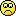Oh well, I cheated a bit. I made it so if my X is less than the grenades it aims at the players left edge and greater for the right edge. Now it hits.... most of the time.   n/a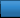AdvertisementWorth A Click